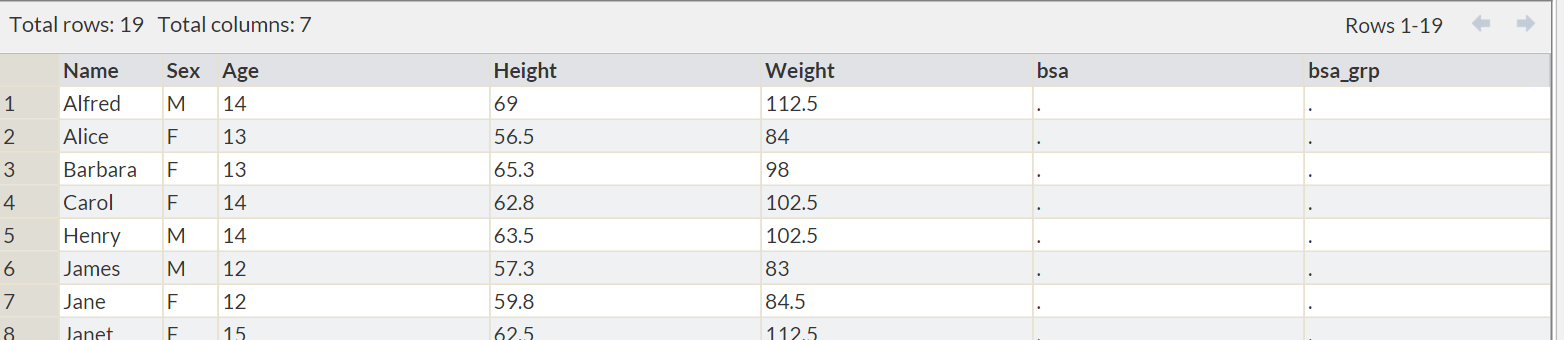## SAS tips & tricks #9 – NOTE: Variable X is uninitialized.

The “NOTE: Variable X is uninitialized” message is a result of trying to run a DATA Step which utilizes a variable that is not present in the input dataset or has not been created at the point within the DATA Step where SAS tries to utilize the variable.

## What causes the message?

This message occurs when a DATA Step  attempts to use a variable which is either not present in the input dataset or has not been created in a preceding step. For example, if the variable was drop or not kept in in the input dataset, or if the code to create it has been accidentally omitted.

## What happens when this occurs?

When SAS encounters an uninitialized variable within a DATA Step, SAS will:

• outputs a message to a log stating the name of the uninitialized variable.
• set the uninitialized variable to missing, i.e. create a null numeric variable.
• continue to create the output dataset.

## How to resolve it?

To resolve this issue, ensure that the uninitialized variable is available in your dataset at the point at which it is first required.

### Example

The following code reads the SASHELP.CLASS dataset and attempts to create a new variable BSA_GRP, based on the value of a variable BSA:

```DATA class;
SET sashelp.class;
IF bsa > 1 THEN bsa_grp = 1;
ELSE IF bsa> 1 THEN bsa_grp = 2;
RUN;```

This code results in the following messages being printed to the log.:

``` NOTE: Variable bsa is uninitialized.
NOTE: There were 19 observations read from the data set SASHELP.CLASS.
NOTE: The data set WORK.CLASS has 19 observations and 7 variables.```

The first line tells us that the variable BSA was uninitialized (not available) at the point in the DATA Step where it was first required. The remaining lines tell us that despite the uninitialized variable, the dataset WORK.CLASS is still created.

We confirm this by opening the output dataset, which will be as follows:We see that SAS has created the output dataset, we also notice that it contains an empty numeric variable BSA.

To resolve this issue, either the BSA variable should be present in the input dataset or else it should be created in the current DATA Step prior to the statement in which the BSA variable is first used, for example:

```DATA class;
SET sashelp.class;
bsa = ((height *weight )/ 3131 )**1/2
IF bsa > 1 THEN bsa_grp = 1;
ELSE IF bsa> 1 THEN bsa_grp = 2;
RUN;```